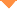首页 > 论文 > Chinese Optics Letters > 18卷 > 12期(pp：12)

Concurrent Photonic Measurement of Angle-of-Arrival and Chirp Rate of Microwave LFM Signal [Early Posting]

• 摘要• 论文信息• 参考文献• 被引情况A photonic approach to concurrently measure the angle-of-arrival (AOA) and the chirp rate of a linear frequency modulated (LFM) signal is proposed and experimentally demonstrated. The measurement is achieved by estimating the differential frequency of a two-tone signal output by a dual-parallel Mach-Zehnder modulator and an additional asymmetry Mach-Zehnder interferometer. Experiments show that the AOA and the chirp rate are measured simultaneously, with an AOA measurement error of $\pm 0.1^\circ$ at an SNR of 9.6 dB. When SNR = -10.4 dB, the AOA error is $\pm 1.3^\circ$ and the chirp rate, measured as $210.2\pm1.5\ \rm{Hz/ps}$ has a standard deviation of $0.7\%$. The measured chirp rate agrees well with the real LFM signal.

Li Shangyuan,Cao Haidong,Xiaoping Zheng. Concurrent Photonic Measurement of Angle-of-Arrival and Chirp Rate of Microwave LFM Signal[J].Chinese Optics Letters,2020,18(12):12.